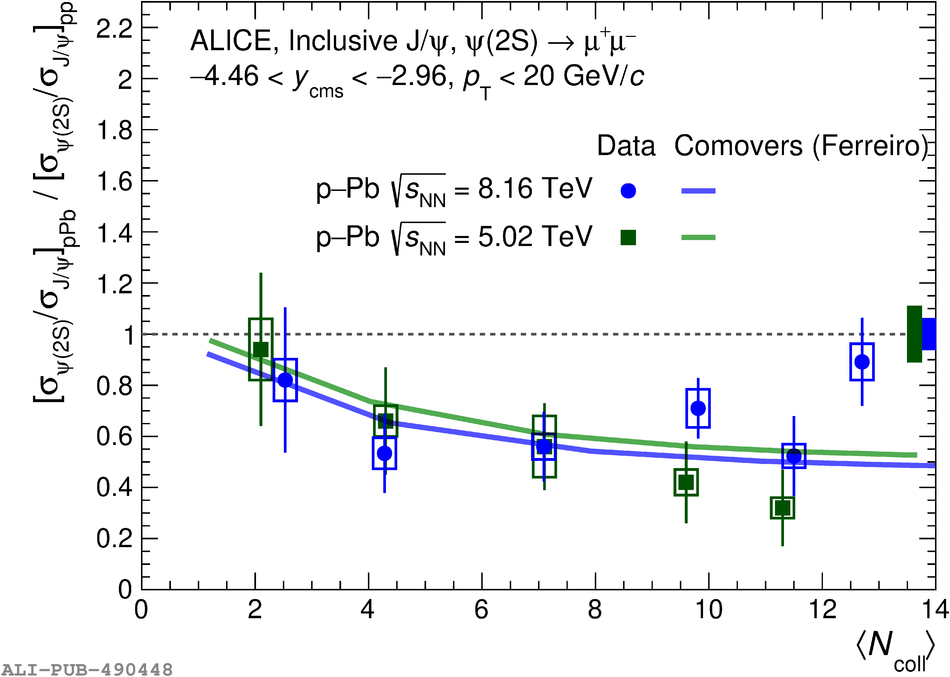# Figure 10

 Double ratio $[\sigma_{\psi(\rm 2S)}/\sigma_{\rm J/\psi}]_{\rm pPb}/[\sigma_{\psi(\rm 2S)}/\sigma_{\rm J/\psi}]_{\rm pp}$ as a function of $\langle N_{\rm coll} \rangle$ at backward (left) and forward (right) rapidity compared with the one at $\sqrt{s_{\rm NN}} = 5.02$ TeV . The vertical error bars represent the statistical uncertainties and the open boxes around the data points the uncorrelated systematic uncertainties. The boxes around unity represent the correlated systematic uncertainty and correspond to the uncertainty on the ratio $\psi(2S)/\jpsi$ in pp collisions. Experimental points are compared with the theoretical predictions of the comovers model at $\sqrt{s_{\rm NN}} = 5.02$ TeV (green line ) and $\sqrt{s_{\rm NN}} = 8.16$ TeV (blue line ).